Author: Oscar Cronquist Article last updated on November 15, 2017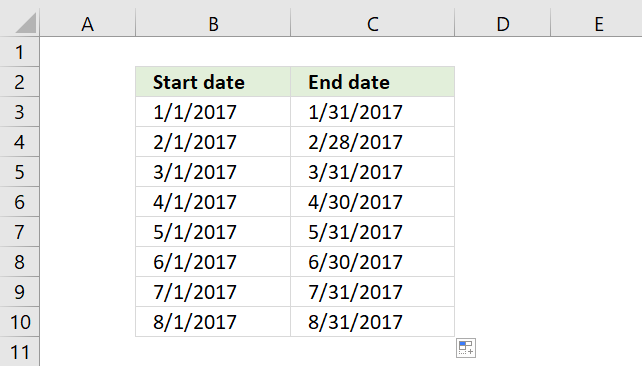I will demonstrate three different techniques to build monthly date ranges in this article. Two of these techniques are easy because they have the start and end date in a cell each.

To have two dates in the same cell makes it more complicated but I have a solution for that, as well.

### Create date ranges using a built-in feature

To build a date range that begins with the first date in a month and ends with the last date in a month follow these steps:

1. Type the start date of your first date range in one cell
2. Type the end date of your first date range in the next cell
3. Repeat above steps to enter the second date range in cells below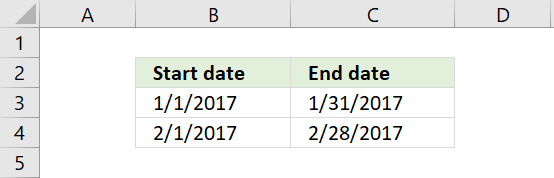4. Select all cells
5. Press and hold on black dot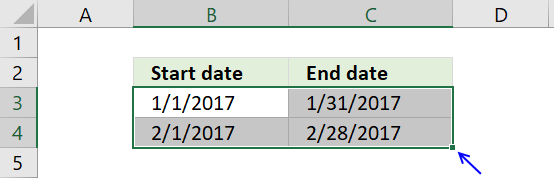6. Drag to cells below as far as neededExcel dates are actually numbers, the above technique uses Excel's ability to quickly create number sequences using the selected cells as a guide to determine the size of the date ranges below.

### Basic formula

Type the start date in a cell, in this case, 1/1/2017. Type the following formula in the next cell:

=DATE(YEAR(B3),MONTH(B3)+1,1)-1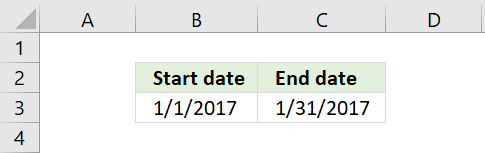The formula in cell C3 calculates the last date for the month and year in cell B3. It actually calculates the first date in the next month and then subtracts with 1.

This solves the issue with some months having 30 days and some having 31 and February with 28 days in common years and 29 days in leap years.

The next date range has the first date in the next month as the start date. The formula is almost identical to the previous formula.

Formula in cell B4:

=DATE(YEAR(B3),MONTH(B3)+1,1)

Now copy cell C3 and paste to cell C4.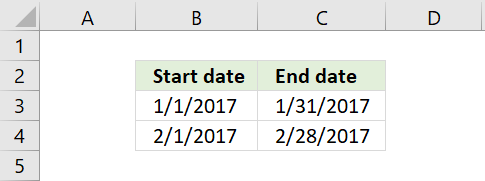The relative cell references in the formula changes accordingly, you can read more about relative and absolute cell references here:

Recommended articles

How to use absolute and relative references
What is a reference in Excel? Excel has an A1 reference style meaning columns are named letters A to XFD […]

Copy cell range B4:C4 and paste to cells below as far as needed.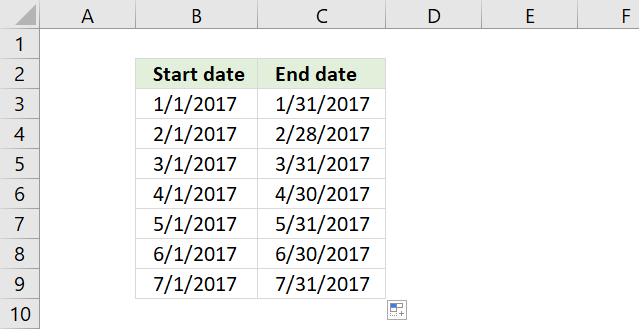The following picture shows you date ranges in a single cell each. To get that you need to build a somewhat more complicated formula.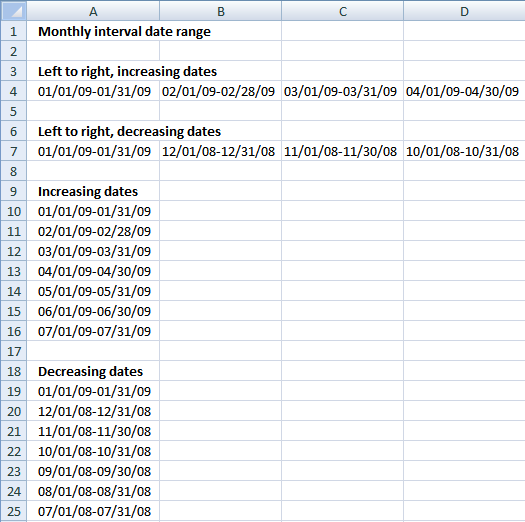### Formula in A4:

=TEXT(DATE(2009,  COLUMN(A:A),  1),  "MM/DD/YY")&"-"&TEXT(DATE(2009, COLUMN(A:A)+1,  1)-1,  "MM/DD/YY") + ENTER

copied right as far as necessary. The TEXT function formats the number from the DATE function as a readable text string in a format you choose. You can read more about the TEXT function here:

Recommended articles

How to use the TEXT function
This article demonstrates how to use the TEXT function in great detail. The formula in cell D3 formats the number […]

### Formula in A7:

=TEXT(DATE(2009,  2-COLUMN(A:A),  1),  "MM/DD/YY")&"-"&TEXT(DATE(2009, 2-COLUMN(A:A)+1,  1)-1,  "MM/DD/YY") + ENTER

copied right as far as necessary.

### Formula in A10:

=TEXT(DATE(2009,  ROW(1:1),  1),  "MM/DD/YY")&"-"&TEXT(DATE(2009, ROW(1:1)+1,  1)-1,  "MM/DD/YY") + ENTER

copied down as far as necessary.

### Formula in A19:

=TEXT(DATE(2009,  2-ROW(1:1),  1),  "MM/DD/YY")&"-"&TEXT(DATE(2009, 2-ROW(1:1)+1,  1)-1,  "MM/DD/YY") + ENTER

copied down as far as necessary.

### Get *.xlsx file

Create a monthly date range.xlsx